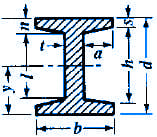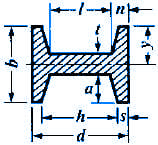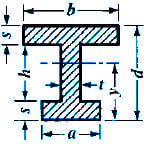Moment of Inertia, Section Modulus, Radii of Gyration Equations
I Sections

Moment of Inertia, Section Modulus, Radii of Gyration Equations I Sections

 A = Area (in2, mm2) I = Moment of Inertia (in4, mm4) Gr = Radius of Gyration = (in, mm) y = Distance of Axis to Extreme Fiber (in, mm)

 SectionOpen Section Properties Case 23 CalculatorOpen Section Properties Case 24 CalculatorOpen Section Properties Case 25 Calculator Moment of InertiaSlope g = (h - l) / (b - t)Radius of GyrationAreaySectionOpen Section Properties Case 26 CalculatorOpen Section Properties Case 27 Calculator Moment of Inertiag = Slope = ( h - l ) / ( b - t )Radius of GyrationAreayRelated:Membership Register | LoginHomeEngineering Book StoreEngineering ForumExcel App. DownloadsOnline Books & ManualsEngineering NewsEngineering VideosEngineering CalculatorsEngineering ToolboxGD&T Training Geometric Dimensioning TolerancingDFM DFA TrainingTraining Online EngineeringAdvertising CenterCopyright Notice